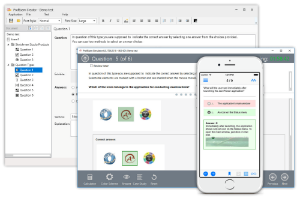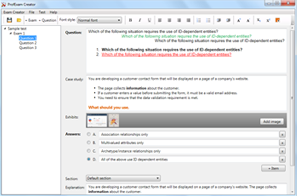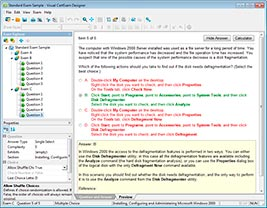# Download Java SE 8 Programmer II.testkings.1z0-809.1e.63q.vcex

## File Info

 Exam Java SE 8 Programmer II Number 1z0-809 File Name Java SE 8 Programmer II.testkings.1z0-809.1e.63q.vcex Size 2.72 Mb Posted April 08, 2019 Downloads 84

### How to open VCEX & EXAM Files?

Files with VCEX & EXAM extensions can be opened by ProfExam Simulator.

Coupon: EXAMFILESCOM
With discount: 20%

## Demo Questions

Question 1
Given the code fragment:
public class Foo {
public static void main (String [ ] args) {
Map<Integer, String> unsortMap = new HashMap< > ( );
unsortMap.put (10, “z”);
unsortMap.put (5, “b”);
unsortMap.put (1, “d”);
unsortMap.put (7, “e”);
unsortMap.put (50, “j”);
Map<Integer, String> treeMap = new TreeMap <Integer, String> (new
Comparator<Integer> ( )  {
@Override public int compare (Integer o1, Integer o2) {return o2.compareTo
(o1); }  } );
treeMap.putAll (unsortMap);
for (Map.Entry<Integer, String> entry : treeMap.entrySet () ) {
System.out.print (entry.getValue () + “ “);
}
}
}
What is the result?

• A: A compilation error occurs.
• B: d b e z j
• C: j z e b d
• D: z b d e j

Question 2
Given:
class Bird  {
public void fly ()  {  System.out.print(“Can fly”); }

class Penguin extends Bird  {
public void fly ()  {  System.out.print(“Cannot fly”); }

and the code fragment:
class Birdie {
public static void main (String [ ] args)  {
fly( ( ) -> new Bird ( ));
fly (Penguin : : new);
}
/* line n1 */

Which code fragment, when inserted at line n1, enables the Birdie class to compile?

• A: static void fly (Consumer<Bird> bird)  {
bird :: fly ();
}
• B: static void fly (Consumer<? extends Bird> bird)  {
bird.accept( ) fly ();
}
• C: static void fly (Supplier<Bird> bird)  {
bird.get( ) fly ();
}
• D: static void fly (Supplier<? extends Bird> bird)  {
LOST

Question 3
Given:
class Sum extends RecursiveAction   {                 //line n1
static final int THRESHOLD_SIZE  = 3;
int stIndex, lstIndex;
int [ ] data;
public Sum (int [ ]data, int start, int end)   {
this.data = data;
this stIndex = start;
this. lstIndex = end;
}
protected void compute ( )    {
int sum = 0;
if (lstIndex – stIndex <= THRESHOLD_SIZE)  {
for (int i = stIndex; i < lstIndex; i++)   {
sum += data [i];
}
System.out.println(sum);
} else {
new Sum (data, stIndex + THRESHOLD_SIZE, lstIndex).fork( );
new Sum (data, stIndex,
Math.min  (lstIndex, stIndex + THRESHOLD_SIZE)
).compute ();
}
}

and the code fragment:
ForkJoinPool fjPool = new ForkJoinPool ( );
int data [ ] = {1, 2, 3, 4, 5, 6, 7, 8, 9, 10}
fjPool.invoke (new Sum (data, 0, data.length));
and given that the sum of all integers from 1 to 10 is 55.
Which statement is true?

• A: The program prints several values that total 55.
• B: The program prints 55.
• C: A compilation error occurs at line n1.
• D: The program prints several values whose sum exceeds 55.

Question 4
Given the content of Operator.java, EngineOperator.java, and Engine.java files:and the code fragment:What is the result?

• A: The Engine.java file fails to compile.
• B: The EngineOperator.java file fails to compile.
• C: The Operator.java file fails to compile.
• D: ON OFF

Question 5
Given the code fragment:
public void recDelete (String dirName) throws IOException   {
File [ ] listOfFiles = new File (dirName) .listFiles();
if (listOfFiles ! = null && listOfFiles.length >0)   {
for (File aFile : listOfFiles)   {
if (aFile.isDirectory ())    {
recDelete (aFile.getAbsolutePath ());
}    else   {
if (aFile.getName ().endsWith (“.class”))
aFile.delete ();
}
}
}

Assume that Projects contains subdirectories that contain .class files and is passed as an argument to the recDelete () method when it is invoked.
What is the result?

• A: The method deletes all the .class files in the Projects directory and its subdirectories.
• B: The method deletes the .class files of the Projects directory only.
• C: The method executes and does not make any changes to the Projects directory.
• D: The method throws an IOException.

Question 6
Given the code fragment:
Map<Integer, String> books = new TreeMap<>();
books.put (1007, “A”);
books.put (1002, “C”);
books.put (1001, “B”);
books.put (1003, “B”);
System.out.println (books);
What is the result?

• A: {1007 = A, 1002 = C, 1001 = B, 1003 = B}
• B: {1001 = B, 1002 = C, 1003 = B, 1007 = A}
• C: {1002 = C, 1003 = B, 1007 = A}
• D: {1007 = A, 1001 = B, 1003 = B, 1002 = C}

Question 7
Given:
class Book {
int id;
String name;
public Book (int id, String name)  {
this.id = id;
this.name = name;
}
public boolean equals (Object obj) {           //line n1
boolean output = false;
Book b = (Book) obj;
if (this.name.equals(b name))}
output = true;
}
return output;
}

and the code fragment:
Book b1 = new Book (101, “Java Programing”);
Book b2 = new Book (102, “Java Programing”);
System.out.println (b1.equals(b2));                 //line n2
Which statement is true?

• A: The program prints true.
• B: The program prints false.
• C: A compilation error occurs. To ensure successful compilation, replace line n1 with:
boolean equals (Book obj)  {
• D: A compilation error occurs. To ensure successful compilation, replace line n2 with:
System.out.println (b1.equals((Object) b2));

Question 8
Given the code fragment:
public static void main (String [ ] args) throws IOException   {
System.out.print (“Enter GDP: “);
//line 1

Which code fragment, when inserted at line 1, enables the code to read the GDP from the user?

• A: int GDP = Integer.parseInt (br.readline());
• B: int GDP = br.read();
• C: int GDP = br.nextInt();
• D: int GDP = Integer.parseInt (br.next());

Question 9
Given:
class Student    {
String course, name, city;
public Student (String name, String course, String city)   {
this.course = course; this.name = name; this.city = city;
}
public String toString()    {
return course + “:” + name + “:” + city;
}
and the code fragment:
List<Student> stds = Arrays.asList(
new Student (“Jessy”, “Java ME”, “Chicago”),
new Student (“Helen”, “Java EE”, “Houston”),
new Student (“Mark”, “Java ME”, “Chicago”));
stds.stream()
.collect(Collectors.groupingBy(Student::getCourse))
.forEach(src, res) -> System.out.println(scr));
What is the result?

• A: [Java EE: Helen:Houston]
[Java ME: Jessy:Chicago, Java ME: Mark:Chicago]
• B: Java EE
Java ME
• C: [Java ME: Jessy:Chicago, Java ME: Mark:Chicago]
[Java EE: Helen:Houston]
• D: A compilation error occurs.

Question 10
Given the code fragments:
interface CourseFilter extends Predicate<String>    {
public default boolean test (String str)     {
return str.equals (“Java”);
}

and
List<String> strs = Arrays.asList(“Java”, “Java EE”, “Java ME”);
Predicate<String> cf1 = s - > s.length() > 3;
Predicate cf2 = new CourseFilter()   {         //line n1
public boolean test (String s)  {
return s.contains (“Java”);
}
};
long c = strs.stream()
.filter(cf1)
.filter(cf2                        //line n2
.count();
System.out.println(c);
What is the result?

• A: 2
• B: 3
• C: A compilation error occurs at line n1.
• D: A compilation error occurs at line n2.

CONNECT US##### PROFEXAM WITH A 20% DISCOUNT

You can buy ProfExam with a 20% discount..

HOW TO OPEN VCEX AND EXAM FILES

Use ProfExam Simulator to open VCEX and EXAM filesHOW TO OPEN VCE FILES

Use VCE Exam Simulator to open VCE files